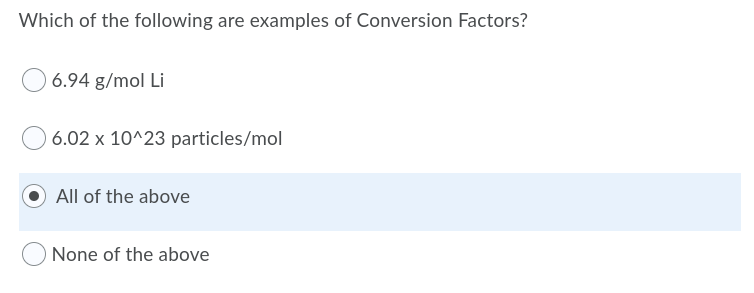# Conversion Factor Question

## Recommended Posts

Hey everyone! Super quick question.

"Is 6.94 g/mol Li a conversion factor?"

I'm just unsure of what the answer could be. I assume it is because you use the molar mass to determine the mass of a substance? Thanks for the help!

##### Share on other sites

52 minutes ago, Kagi98JP said:

Hey everyone! Super quick question.

"Is 6.94 g/mol Li a conversion factor?"

I'm just unsure of what the answer could be. I assume it is because you use the molar mass to determine the mass of a substance? Thanks for the help!

Conversion of what to what ?

The molar mass of Lithium is 6.941 grammes per mole.

Do you understand what this means ?

##### Share on other sites

3 minutes ago, studiot said:

Conversion of what to what ?

The molar mass of Lithium is 6.941 grammes per mole.

Do you understand what this means ?

I understand what it means. I just don't understand whether or not 6.941 g/mol is a conversion factor. The question is worded abhorrently.

For example, 6.02 x 10^23 mol^-1 would be a conversion factor, but is 6.941 g/mol one? You use the molar mass of Li (g/mol) to determine either the mass (g) or amount of moles (m), right? So is that an example of a conversion factor? A value, paired with 2 units (in this case g/mol) that can determine the value of another unit?

##### Share on other sites

Well I wouldn't call any of these conversion factors.

Here is an example of what I would call a conversion factor.

Convert 8 kilometers to miles.

the conversion factor is 5/8 and it is multiplicative (you multiply what you have by it to get what you want.)

So 8 kilometers is 8 x 5/8 = 5 miles.

So what were the exact words of the question ?

Edited by studiot
##### Share on other sites

This is the exact question:I'm fairly certain 6.02 x 10^23 mol^-1 (Avogadro's constant) is a conversion factor.

##### Share on other sites

Here is a very strong hint.

In my example both what you have and what you want to convert to measure the same thing or quantity.

All you are doing is 'converting' from one unit to another.

So in my exmaple you are measuring distance ( 8 kilometres) and you want that distance in miles.

So you apply the conversion factor for kilometers to miles.

You might like to try asking google for convertion factors for pints to litres, pounds to kilogrammes, dollars to Euros etc and see what the results are.

You cannot turn apples into donkeys by means of a conversion factor.

Although one famous mathematician defined a Mathematician as a machine for converting coffee into theorems.

Edited by studiot
##### Share on other sites

16 minutes ago, Kagi98JP said:

This is the exact question:

I'm fairly certain 6.02 x 10^23 mol^-1 (Avogadro's constant) is a conversion factor.

I agree it seems a strange* question in some ways but I found this which seems to help: https://www.thoughtco.com/definition-of-conversion-factor-604954

So it says a conversion factor is between different units for the same quantity. In the case of the mole, it is a unit for numbers of particles, expressing them in more convenient form for use in chemistry, so I think I would agree Avogadro's number can be considered a conversion factor.

But grams to moles?........

*actually damned silly , in my view. What's the point of tripping students up with pedantry like this, that has no impact on their understanding of physical science? Just puts students off, in my view. But don't tell anyone I said that.

Edited by exchemist

## Create an account or sign in to comment

You need to be a member in order to leave a comment

## Create an account

Sign up for a new account in our community. It's easy!

Register a new account